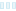# Statistical analysis of a medical dataset and create at least 3 tables

Freelancer: cinziazullian
Winner
Runner UpRejected

## tatistical analysis of a medical dataset

To conduct this statistical analysis of the provided medical dataset, I follow the following steps: Summarize the baseline data (labeled with 1) by calculating the mean and standard deviation for continuous variables and counts and percentages for categorical variables. Calculate P-values for all comparisons of the three groups using a suitable statistical test, such as the ANOVA test or the chi-squared test. Create a table that describes the distribution of variables X, Y, Z, and AA versus variables BB, BC, BD, and AB according to the outcome (column AF). Calculate the means and standard deviations for continuous variables and the counts and percentages for categorical variables for each outcome group. Use a regression analysis to identify the independent predictors of the outcome (column AF). Specifically, determine whether some of the baseline data (labeled with 1) predict an outcome of 1 (worsened) or 2 in column AF. Calculate P-values for each independent predictor.

Entry #12

No messages yet.